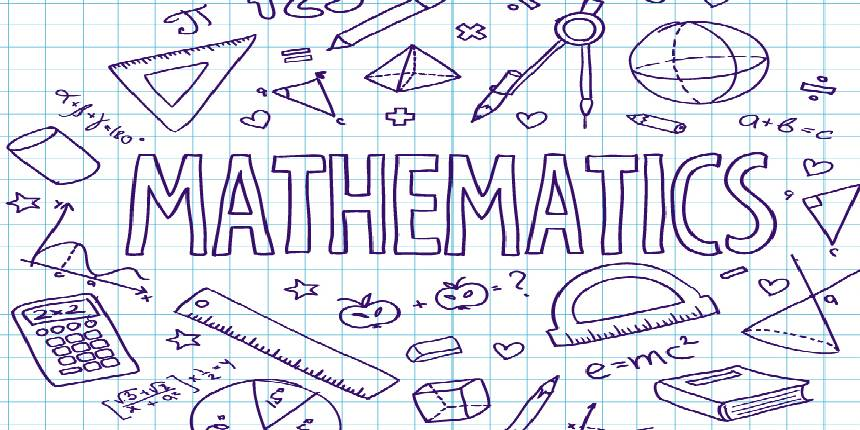NCERT Books for Class 12 Maths: NCERT Books are designed under the National Curriculum Framework. Schools affiliated to CBSE prescribe the NCERT books. Students can take the help of these NCERT Maths books to know about the Class 12th syllabus and study accordingly. Hence, students are advised to study from NCERT Class 12th Maths book to understand the course well and score high. These books contain all topics and chapters that are covered in CBSE class 12 Syllabus for Maths in the simplest manner so that students can easily understand the same. Students should study all the subjects from NCERT books as these are the best for the better board exam and KVPY, JEE, NEET competitive exam preparation. In this article, we will discuss in detail about the NCERT Maths book for Class 12 and their importance.

Importance of using NCERT books for class 12th Maths:

• NCERT books are written by the experts after detailed research and thus these books help in giving accurate knowledge to students.

• Every topic, mentioned in these books, is written in simple language for a better understanding of students.

• NCERT Maths books play a major role in clearing the fundamental concepts of the students.

• Students can score high with the help of these NCERT books for Class 12th Maths as they strictly follow the CBSE curriculum

• The question papers of CBSE Class 12th board examinations are formed by referring the NCERT Maths books. Hence, students can treat the given exercises as sample questions for board exams.

How to Download NCERT Book for Class 12 Maths Pdf Free?

Candidates who want to access the free pdf of NCERT maths books for class 12 can either click the below-given link or can visit the official website of NCERT to download the free NCERT book pdf. Follow the below-given steps to download the same:

• Click on the respective chapter link or visit the official website http://ncert.nic.in/textbook/textbook.htm

• Select the desired subject and class

• A pdf file will open on your computer screen.

• Click on the arrow provided above to download the free pdf file of NCERT Class 12 Maths book

How to buy NCERT Class 12 Maths books Online?

Follow the mentioned steps to purchase the NCERT Class 12 Maths book online-

• Students can visit the official website of NCERT, https://ncertbooks.ncert.gov.in/login to order the Class 12 Maths Books online

• Register online by providing the basic info before placing the order.

• Now, Click on the link "order textbooks online" and add the Class 12 Maths NCERT books in the cart

• Select the “proceed to payment” for paying the amount through online mode by using net banking, credit card or debit card.

NCERT Book for Class 12 Maths:

 NCERT Books Chapters Mathematics Part - I PrelimsChapter 1: Relations and FunctionsChapter 2: Inverse Trigonometric FunctionsChapter 3: MatricesChapter 4: DeterminantsChapter 5: Continuity and DifferentiabilityChapter 6: Application of DerivativesAnswersAppendix - IAppendix - II Mathematics Part - II PrelimsChapter 7: IntegralsChapter 8: Application of IntegralsChapter 9: Differential EquationsChapter 10: Vector AlgebraChapter 11: Three Dimensional GeometryChapter 12: Linear ProgrammingChapter 13: ProbabilityAnswers

Best Reference Books for Class 12 Maths:

Students should use study material as references during the preparation for the board exams. This will help them to stay on an organized schedule. Hence, it is important to have access to the best books for CBSE Class 12th Maths. Simply mugging up the NCERT Book for Class 12 Maths won’t be sufficient for the preparation. Students should be thorough with the NCERT books at least 6 months before 12th boards. This will give them enough time to consider using other reference books. Some of the best reference books for CBSE 12 Maths are listed below:

1. CBSE Chapter-wise Solutions – Mathematics (Class 12) by Hemant Malhotra

2. CBSE Examination Mathematics: 15 Sample Question Papers (Class 12) by Prem Kumar

3. Mathematics (Class – XII) by NCERT

4. Problems Plus In IIT Mathematics by A Das Gupta

5. Senior Secondary School Math 12 by R. S. Aggarwal

6. Solutions of Objective Mathematics: Solutions of Exercises and Chapter Tests by Dhanpat Rai

How to Prepare from NCERT Book for Class 12 Maths to Score High?

• Students should finish the CBSE syllabus of 12th grade based on the CBSE Class 12th marking scheme of each unit.

• Units with high weightage of Maths NCERT class 12th should be thoroughly prepared. At the same time, students must have a basic knowledge of all the chapters.

• Solve CBSE class 12th question papers of all types. If the candidates are preparing for JEE or NEET, they can solve JEE practice questions and NEET practice questions as well. These questions include practice questions from the NCERT Book for Class 12 Maths.

• Students can refer to the NCERT Maths solution while practicing questions.

Never miss exam and college updates
Top
The question have been saved in answer later, you can access it from your profile anytime. Access now